# ES - Editorial

Author: Full name
Tester: Full name
Editorialist: Oleksandr Kulkov

MEDIUM-HARD

None

### PROBLEM:

You have to calculate \sum\limits_{k=1}^n \lfloor k\cdot e\rfloor with n \leq 10^{4000}.

### QUICK EXPLANATION:

See the pictures below for n=6.

### EXPLANATION:

Geometrically, we have to count lattice points with 0 < y \leq ex. Let’s add to the answer points below y=\lfloor e\rfloor x = 2x. There are \lfloor e \rfloor \cdot \dfrac{n(n+1)}{2}=n(n+1) of them. Now there is one to one correspondence between remaining points and points below y=(e-\lfloor e \rfloor)x. Let’s transform plane in such a way that (n, \lfloor(e-\lfloor e \rfloor)n\rfloor) point will move to (0,0). Now we have to count lattice points below y=\dfrac{x+(e-\lfloor e \rfloor)n-\lfloor(e-\lfloor e \rfloor)n\rfloor}{e-\lfloor e \rfloor}, thus we returned to the task of counting points below y=kx+b with k>1. Let’s see how it works for n=6 and proceed to the formal algorithm.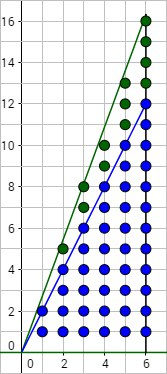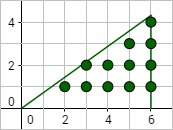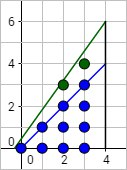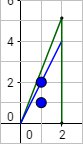Now assume we want to count lattice (x;y) such that 0 \le x < n and 0 < y \le kx+b. Which is \sum\limits_{x=0}^{n-1}\lfloor kx+b \rfloor.

1. If k>1 or b>1, we can count points with y\le\lfloor k\rfloor x + \lfloor b\rfloor which is \sum\limits_{t=0}^{n-1} \lfloor k \rfloor t + \lfloor b \rfloor=\dfrac{(\lfloor k\rfloor (n-1) + 2 \lfloor b \rfloor)n}{2}. Now we have to count points in \lfloor k \rfloor x + \lfloor b \rfloor < y\leq kx+b which is same as counting points in 0< y\leq (k-\lfloor k \rfloor)x+(b-\lfloor b \rfloor). Thus k'=k-\lfloor k \rfloor < 1, b' = b-\lfloor b \rfloor < 1.
2. If k < 1 and b < 1 then considering that there are no lattice points in x< 0, 0< y\le kx+b because b < 1 and in x< n, \lfloor k n + b \rfloor < y\le kx+b, we can rotate the trapezium in such way that new origin is situated in (n;\lfloor k n + b \rfloor), y-axis moves to the left and x-axis moves to the bottom, as you may see on the picture: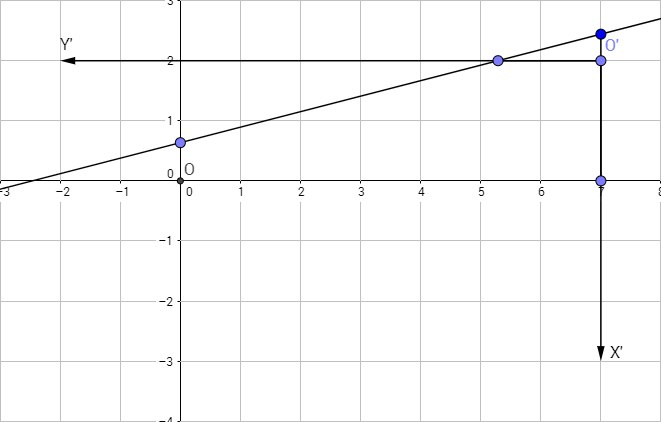One can see that now we deal with 0\leq x < \lfloor kn+b\rfloor and 0< y\le \dfrac{x+(kn+b)-\lfloor kn+b\rfloor}{k} which refers us to the case 1 since k'>1.

Let’s do some complexity analysis now. We assume b to be negligible compared to kn since we can get b< 1 at the first step of case 1. In that case there are at most \dfrac{kn(n-1)}{2} points we have to count and we getting rid of \dfrac{\lfloor k \rfloor n(n-1)}{2} of them. So part of removed points is at least \dfrac{\lfloor k \rfloor}{k}\geq \dfrac 1 2. Thus this solution works in O(\log n) steps. You have to calculate euler’s number with high precision in this problem which is possible using continued fractions or series e=\sum\limits_{n=0}^\infty\dfrac{1}{n!}. Please observe the details in the following link.

Another solution based on Beatty theorem can be found here. And another one solution is based on this article.

### AUTHOR’S AND TESTER’S SOLUTIONS:

Author’s solution can be found here.
Tester’s solution can be found here.

### RELATED PROBLEMS:

@admin This XML file does not appear to have any style information associated with it. The document tree is shown below.